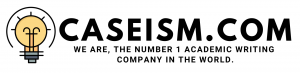# A “Compelling And Pre-Emptive” Offer For The Valspar Corporation Case Solution & Answer

## A “Compelling And Pre-Emptive” Offer For The Valspar Corporation Case  Solution

### Case Overview

The CEO of the Valspar Corporation was considering the merger of his company with a large industry player in 2015. The CEO had a meeting with a key industry competitor, to discuss about the strategic combination of the two companies.But as the industry grew; Valspar realized that it needs to be acquired and it should have a strategic collaboration with Sherwin William. The management at Sherwin William came with an offer price of \$106, but the management was concerned about a fair price to be paid for Valspar’s stock. On the other hand, Valspar had been waiting for a “compelling and a preemptive” offer from Sherwin William,but still there were several question to be answered, i.e. what should be the maximum bidding price and what was probability of the deal to be blocked by the antitrust regulators, ultimately leading towards wastage of time and resources?

### Question 1

The income statement has been calculated as per the given assumptions of sales growth rate of 3%, while the COGS, SG&A, R&D, depreciation and interest expense are taken as the percentage of sales in the year 2015. The restructuring charges, other expenses and gain on sale of assets are kept zero, which resulted in the net income of \$457,526 in 2016 to \$514,949 in 2020. The working capital are forecasted as the 14% of the sales from 2016-2020. The change in working capital is calculated by subtracting the previous year capital from the current year working capital. The capital expenditures are assumed to be equal to the depreciation expense of each year. Moreover, in Valspar; WACC is calculated as 7.88%. The cost of debt is calculated by dividing the interest expense with the long term debt in year 2015. The cost of equity is calculated through CAPM equation, by taking the risk free rate of a 10 year Treasury bond, market risk premium of 5.8% in the USA market in 2015 and the Valspar’s beta of 1.24. The weight of equity and debt are determined by dividing the market value of debt and equity in 2015, with total market value of company’s capital.Lastly, the free cash flows of the firm are calculated and discounted at the WACC of 7.88%, which have resulted in the enterprise value of 8293519.78, out of which the debt is subtracted and divided by the number of shares outstanding in 2015. The resulted share price through DCF valuation is \$78.08.

### Question 2

The income statement has been calculated as per the given assumptions of sales growth rate of 3%, while the COGS, SG&A, R&D, depreciation and interest expense are taken as a percentage of sales in the year 2015. But here, the sales are assumed to have synergies if the company gets acquired, resulting in an increase in sales, as shown in the table. The restructuring charges, other expenses and gain on sale of assets are kept zero, which have resulted in the net income of \$665,852.49 in 2016 to \$633,340.94 in 2020. The working capital are forecasted as 14% of the sales from 2016-2020. The change in working capital is calculated by subtracting the previous year from the current year working capital. The capital expenditures are assumed to be equal to the depreciation expense of each year. Moreover, the Sherwin William’s WACC is calculated as 8.03%. The cost of debt is calculated by dividing the interest expense with the long term debt in year 2015. The cost of equity is calculated through CAPM equation, by taking the risk free rate of a Treasury 10 year bond, market risk premium of 5.8% in the USA market, in 2015, and the Valspar’s levered beta of 1.056. The weight of equity and debt are determined by dividing the market value of debt and equity in 2015, with total market value of company’s capital. Lastly, the free cash flows of the firm are calculated and discounted at the WACC of 8.03%, which have resulted in the enterprise value of \$11654010.3, out of which, the debt is subtracted and divided by the number of shares outstanding in 2015. The resulted share price through DCF valuation is \$118.84.……………………………..

This is just a sample partial case solution. Please place the order on the website to order your own originally done case solution.